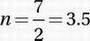# SAT Math Multiple Choice Question 665: Answer and Explanation

### Test Information

Question: 665

5. If 22n-2 = 32, what is the value of n?

• A. 2.0
• B. 2.5
• C. 3.0
• D. 3.5

Explanation:

D

Algebra (exponentials) EASY

22n - 2 = 32

When dealing with exponential equations, it helps to see if we can express the two sides of the equation in terms of the same base. Since 32 = 25, we can express both sides in base 2:

22n - 2 = 25

If xa = xb and x > 1, then a = b (if the bases are equal, the exponents are equal):

2n - 2 = 5